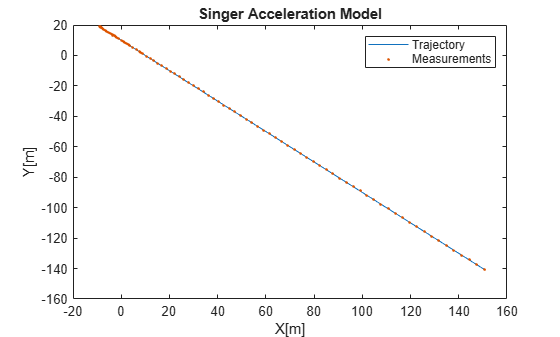# singer

Singer acceleration motion model

## Syntax

``updatedstates = singer(states)``
``updatedstates = singer(states,dt)``
``updatedstates = singer(states,dt,tau)``

## Description

example

````updatedstates = singer(states)` returns the updated states from the current `states` based on the Singer acceleration motion model. The default time step is 1 second.```
````updatedstates = singer(states,dt)` specifies the time step, `dt`, in seconds.```
````updatedstates = singer(states,dt,tau)` specifies the target maneuver time constant, `tau`, in seconds. The default target maneuver time constant is 20 seconds.```

## Examples

collapse all

Define a state matrix for a 2-D Singer acceleration motion.

`states = [1 2 2.5;1 2.5 3;0 -1 2;2 3 -1;5 0 3;-2 4 2];`

Predict the states by using a default time step interval `dt` = 1 second.

`states = singer(states)`
```states = 6×3 2.0000 4.0082 6.4835 1.0000 1.5246 4.9508 0 -0.9512 1.9025 6.0165 4.9671 2.9835 3.0492 3.9016 4.9508 -1.9025 3.8049 1.9025 ```

Predict the state by using `dt` = 0.1 second.

`states = singer(states,0.1)`
```states = 6×3 2.1000 4.1559 6.9881 1.0000 1.4297 5.1406 0 -0.9465 1.8930 6.3119 5.3762 3.4881 2.8594 4.2812 5.1406 -1.8930 3.7859 1.8930 ```

Define a state vector for a 2-D Singer acceleration motion.

```state = [10;-10;3;0;10;-3]; dt = 0.2; % time step in seconds tau = 10; % maneuver time in seconds```

Use the `singer` function to create a trajectory and measure the positions using the `singermeas` function.

```positions = zeros(2,100); % Pre-allocate memory measurements = zeros(3,100); % Pre-allocate memory for i = 1:1:100 state = singer(state, dt, tau); positions(:,i) = [state(1); state(4)]; measurements(:,i) = singermeas(state); end```

Visualize the results.

```plot(positions(1,:), positions(2,:)) hold on plot(measurements(1,:), measurements(2,:), '.') title('Singer Acceleration Model'); xlabel('X[m]'); ylabel('Y[m]'); legend('Trajectory', 'Measurements'); ```## Input Arguments

collapse all

Current states, specified as a real-valued 3N-by-1 vector or a real-valued 3N-by-M matrix. N is the spatial degree of the state, and M is the number of states.

The state vector in each column takes different forms based on its spatial dimensions.

Spatial DegreesState Vector Structure
1-D`[x;vx;ax]`
2-D`[x;vx;ax;y;vy;ay]`
3-D`[x;vx;ax;y;vy;ay;z;vz;az]`

For example, `x` represents the x-coordinate, `vx` represents the velocity in the x-direction, and `ax` represents the acceleration in the x-direction. If the motion model is in one-dimensional space, the y- and z-axes are assumed to be zero. If the motion model is in two-dimensional space, values along the z-axis are assumed to be zero. Position coordinates are in meters. Velocity coordinates are in meters/second. Acceleration coordinates are in m/s2.

Example: `[5;0.1;0.01;0;-0.2;-0.01;-3;0.05;0]`

Time step, specified as a positive scalar in seconds.

Example: `0.5`

Target maneuver time constant, specified as a positive scalar or an N-element vector of scalars in seconds. N is the spatial degree of the state. When specified as a vector, each element applies to the corresponding spatial dimension.

Example: `30`

## Output Arguments

collapse all

Updated states, returned as a real-valued 3N-by-1 vector or a real-valued 3N-by-M matrix. N is the spatial degree of the state, and M is the number of states. The `updatedStates` output has the exactly same form as the `states` input.

## Algorithms

The Singer acceleration model assumes the acceleration at time step k+1, which depends on the acceleration at time step k with exponential decay as:

`$a\left(k+1\right)=a\left(k\right)*\mathrm{exp}\left(-T/\tau \right)$`

where a(k) is the acceleration at time step k, T is the time step, and τ is the target maneuver time constant.

For a 1-D singer model state p = [x, vx, ax]T, the state propagation is:

`$p\left(k\right)=\left[\begin{array}{ccc}1& T& \left(\alpha T-1-{e}^{-\alpha T}\right)/{\alpha }^{2}\\ 0& 1& \left(1-{e}^{-\alpha T}\right)/\alpha \\ 0& 0& {e}^{-\alpha T}\end{array}\right]p\left(k\right)+w\left(k\right)$`

where α = 1/τ is the reciprocal of the target maneuver time constant and w(k) is the Singer model process noise at time step k. See `singerProcessNoise` for more details on the process noise.

 Singer, Robert A. "Estimating optimal tracking filter performance for manned maneuvering targets." IEEE Transactions on Aerospace and Electronic Systems 4 (1970): 473-483.

 Blackman, Samuel S., and Robert Popoli. "Design and analysis of modern tracking systems." (1999).

 Li, X. Rong, and Vesselin P. Jilkov. "Survey of maneuvering target tracking: dynamic models." Signal and Data Processing of Small Targets 2000, vol. 4048, pp. 212-235. International Society for Optics and Photonics, 2000.